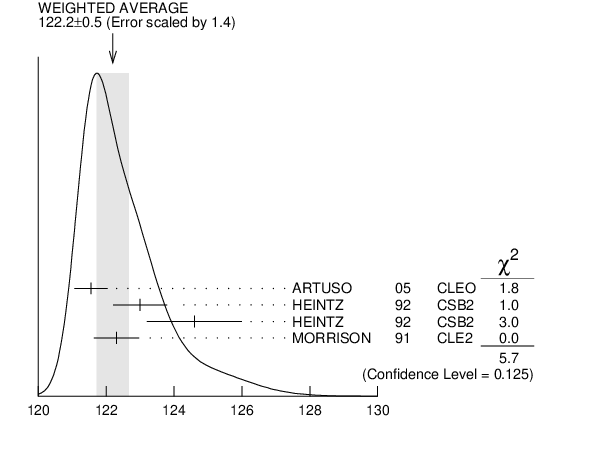# ${{\boldsymbol \gamma}}$ ENERGY IN ${{\boldsymbol \Upsilon}{(3S)}}$ DECAY INSPIRE search

VALUE (MeV) EVTS DOCUMENT ID TECN  COMMENT
$\bf{ 121.9 \pm0.4}$ OUR EVALUATION  Treating systematic errors as correlated
$\bf{ 122.2 \pm0.5}$ OUR AVERAGE  Error includes scale factor of 1.4.
$121.55$ $\pm0.16$ $\pm0.46$
 2005
CLEO ${{\mathit \Upsilon}{(3S)}}$ $\rightarrow$ ${{\mathit \gamma}}{{\mathit X}}$
$123.0$ $\pm0.8$ 4959 1
 1992
CSB2 ${{\mathit e}^{+}}$ ${{\mathit e}^{-}}$ $\rightarrow$ ${{\mathit \gamma}}$ X
$124.6$ $\pm1.4$ 17 2
 1992
CSB2 ${{\mathit e}^{+}}$ ${{\mathit e}^{-}}$ $\rightarrow$ ${{\mathit \ell}^{+}}{{\mathit \ell}^{-}}{{\mathit \gamma}}{{\mathit \gamma}}$
$122.3$ $\pm0.3$ $\pm0.6$ 9903
 1991
CLE2 ${{\mathit e}^{+}}$ ${{\mathit e}^{-}}$ $\rightarrow$ ${{\mathit \gamma}}$ X
1  A systematic uncertainty on the energy scale of 0.9$\%$ not included. Supersedes NARAIN 1991 .
2  A systematic uncertainty on the energy scale of 0.9$\%$ not included. Supersedes HEINTZ 1991 .${{\mathit \gamma}}$ energy in ${{\mathit \Upsilon}{(3S)}}$ decay (MeV)
References:
 ARTUSO 2005
PRL 94 032001 Photon Transitions in ${{\mathit \Upsilon}{(2S)}}$ and ${{\mathit \Upsilon}{(3S)}}$ Decays
 HEINTZ 1992
PR D46 1928 ${\mathit {\mathit b}}{\mathit {\overline{\mathit b}}}$ Spectroscopy from the ${{\mathit \Upsilon}{(3S)}}$ State
 MORRISON 1991
PRL 67 1696 Inclusive ${{\mathit \chi}{(2P)}}$ Production in ${{\mathit \Upsilon}{(3S)}}$ Decay